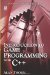# 7.6 Multiple Choice

## 7.6 Multiple Choice

Often it is required to process many possible conditions, and each condition needs a specific response. For example, say a nuclear power station has a number of codes to designate the safety status of the working environment. A code 0 is normal, meaning there is no danger of an explosion. 1 means the status is considered high but there is no impending danger. 2 means the station should be evacuated. Finally, 3 means an explosion has occurred and you should already be dead. One way to process these codes is the following:

`      if(Code == 0)      {         std::cout<<"Code normal\n";      }      if(Code == 1)      {         std::cout<<"Code high\n";      }      if(Code == 2)      {         std::cout<<"Code Danger\n";      }      if(Code == 3)      {         std::cout<<"Code You Should Be Dead\n";      } `

This code is correct and will work, but it isn't easy to read and is tedious to write. The If Else statements are useful when a small number of conditions need to be processed, but for a greater number of conditions, the Switch-Case statement can be used.

### 7.6.1 Switch-Case Statement

The Switch-Case statement can be used to divert a program into many different branches based upon a number of different conditions. It can be thought of as consecutive If statements, as featured earlier in this chapter. It takes the following form:

• Switch(Value)

• Case x: do something

• Case y: do something

• Case z: do something

Thus, the Switch statement is useful for processing many conditions. The switch keyword precedes the variable in the parentheses. This variable is the subject of the statement, and its value remains the matter in question for the rest of the Switch statement. The case keyword is used to question the values of the subject. If the value of the subject matches that of the Case statement, then the code associated with that case is performed; otherwise it is ignored. Consider the following sample C++ code:

`      switch(Code)      {         case 0:         std::cout<<"Code normal\n";         break;         case 1:         std::cout<<"Code high\n";         break;         case 2:         std::cout<<"Code Danger\n";         break;         case 3:         std::cout<<"Code You Should Be Dead\n";         break;      } `Introduction to Game Programming with C++ (Wordware Game Developers Library)
ISBN: 1598220322
EAN: 2147483647
Year: 2007
Pages: 225
Authors: Alan Thorn

Similar book on Amazon

flylib.com © 2008-2017.
If you may any questions please contact us: flylib@qtcs.net